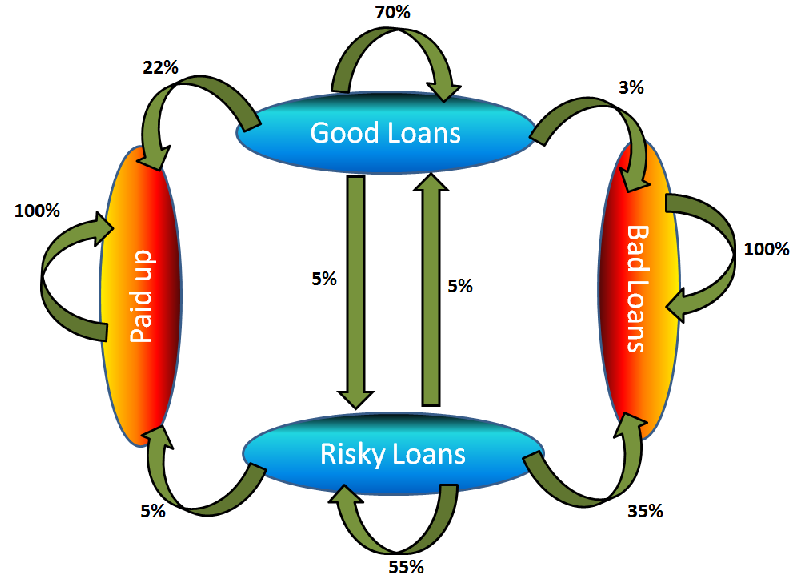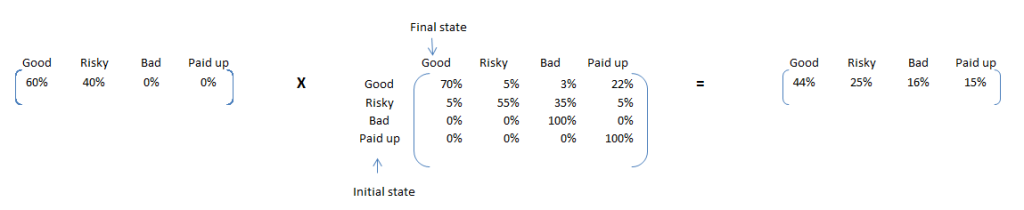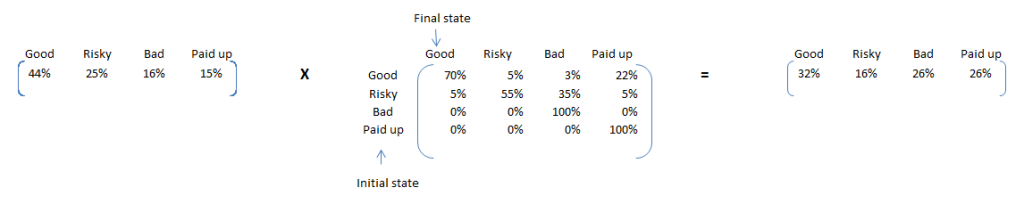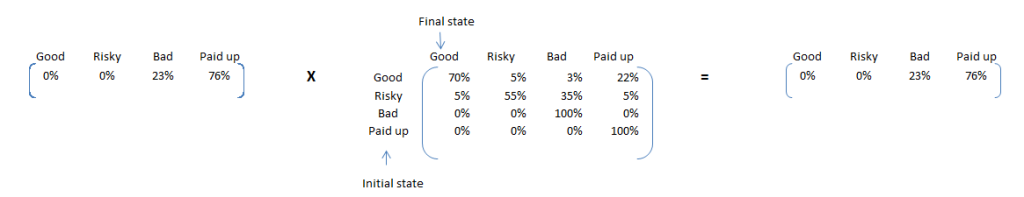Home » Solve a business case using simple Markov Chain

# Solve a business case using simple Markov Chain

Markov process fits into many real life scenarios. Any sequence of event that can be approximated by Markov chain assumption, can be predicted using Markov chain algorithm. In the last article, we explained What is a Markov chain and how can we represent it graphically or using Matrices. In this article, we will go a step further and leverage this technique to draw useful business inferences.

“Krazy Bank”, deals with both asset and liability products in retail bank industry. A big portfolio of the bank is based on loans. These loans make the majority of the total revenue earned by the bank. Hence, it is very essential for the bank to find the proportion of loans which have a high propensity to be paid in full and those which will finally become Bad loans. “Krazy Bank” has hired you as a consultant to come up with these scoping numbers.

All the loans, which have been issued by “Krazy Bank” can be classified into four categories :

1. Good Loans : These are the loans which are in progress but are given to low risk customers. We expect most of these loans will be paid up in full with time.
2. Risky loans : These are also the loans which are in progress but are given to medium or high risk customers. We expect a good number of these customers will default.
3. Bad loans : The customer to whom these loans were given have already defaulted.
4. Paid up loans : These loans have already been paid in full.

Short Note on Absorbing nodes

Absorbing nodes in a Markov chain are the possible end states. All nodes in Markov chain have an array of transitional probability to all other nodes and themselves. But, absorbing nodes have no transitional probability to any other node. Hence, if any individual lands up to this state, he will stick to this node for ever. Let’s take a simple example. We are making a Markov chain for a bill which is being passed in parliament house. It has a sequence of steps to follow, but the end states are always either it becomes a law or it is scrapped. These two are said to be absorbing nodes. For the loans example, bad loans and paid up loans are end states and hence absorbing nodes.

Transition diagram

You have done a thorough research on past trends of loan cycle and based on past trends here is the Markov chain you observed (for a period of 1 year):Explaining absorbing nodes becomes simple from this diagram. As you can see, Paid up and bad loans transition only to themselves. Hence, whatever path a process takes, if it lands up to one of these two states, it will stay there for ever. In other words, a bad loan cannot become paid up, risky or good loan ever after. Same is true with paid up loans.

Transition calculations

Once, we have  1 year transition probability, we can convert prediction algorithm to simple matrix multiplication. Currently the portfolio has 60% Good and 40% Risky loans. We look forward to calculate, how many of these loans will be finally paid up in full? Using 1 year transition probability, we can estimate the number of loans falling into each of the four bucket 1 year down the line.Here are some interesting insights from this calculation. We can expect 15% of the loans to be paid up in this year and 16% being ending up as bad loans. As the % of bad loans seem to be on a higher side, it will be beneficial to identify these loans and make adequate interventions. Pin pointing to these 15% is not possible using simple Markov chain, but same is possible using a Latent Markov model.

Now, to make a prediction for 2 years, we can use the same transition matrix. This time the initial proportions will the final proportions of last calculation. Transition probability generally do not change much. This is because, it is based on several time points in past.If we keep on repeating this exercise, we see the proportion matrix converges. Following is the converged matrix. Note that, multiplying it with transition matrix makes no change to proportions.These are the proportion numbers we were looking for. 54% of the current loans will be paid up in full but 46% will default. Hence doing this simple exercise lead us to such an important conclusion that the current portfolio exposes bank to a very high risk.

Loan transition deep dive

We have already seen the stationary point proportions for the portfolio. Something which will be of interest to us next is that what proportion of Good loans land up being paid up in full. For this we can start with an initial proportion split of Good – 100% and rest – 0%. The final converged matrix is as follows:Here are some interesting insights. If the entire portfolio was built of good loans, only 23% of loans would have defaulted against 46% for current portfolio. Hence, we will expect a very high proportion of risky loans will show default. We can find this using a simple transition calculation using Risky – 100% and rest – 0%. Following is the final converged matrix :80% of such loans will default. Hence, our classification of Risky and Good separates out propensity to default pretty nicely.

End Notes

In this article, we saw how Markov chain can be used to find out multiple insights and make good predictions on an overall level. There are many other processes which can be explained using Markov chain. In such cases, Markov chain algorithm will give you number of insights and will serve as a very handy forecasting tool. However, Markov chain can only make forecast on segment level and not make prediction on customer level. In case you need to make customer level forecast, you need a Latent Markov model and not a simple Markov model.

Did you find the article useful? Are you aware of any other real life Markov process? Can Markov chain be used in that process to bring out interesting insights? Did this article solve any of your existing problems? Have you used simple Markov chain in any other context ?

### If you like what you just read & want to continue your analytics learning, subscribe to our emails, follow us on twitter or like our facebook page.

You can also read this article on our Mobile APP•pradeep says:

Hi Tavish,

Thank you for sharing this. It’s interesting and very useful. I have one clarification how do we get the “Final state transition matrix” 4×4 matrix?.

Sorry if i am missing something here.

Regards,

•Tavish Srivastava says:

The case discussed in this article has a constant transition probability matrix. Do you can use the same matrix throughout.

Tavish

•Koushik Kar says:

Hi Tavish,

I have recently enrolled for a course in BA and trying to grasp as much information as possible. This Markov Chain problem correlates with some of the current issues in my Organization. Waiting for your future publications.

Thanks & Regards,

Koushik

•Tavish Srivastava says:

Koushik,

Can you illustrate the problem you are referring to?

•Koushik Kar says:

I work for a Facility Management Company and the biggest challenge for the company is to maintain the cash flow due to large no of client base and payments not coming in time . Trying to figure out how I can help them in this situation.

•uday says:

Tavish
this is interesting. can you elaborate on the deeep dive section.. how you arrived at the final converged matrix

You mention “For this we can start with an initial proportion split of Good – 100% and rest – 0%. ”
So would the initial matrix be
[100 0 0 0]

If that is the initial matrix how do you arrive at
[0 0 23 76]

Thanks for your papers. they are very good for novices like me.
Uday

•Tavish Srivastava says:

Uday,

The converged matrix is found by multiplying the transition matrix many times to the the current state. This is basically the final proportion of state distribution. You are right the initial matrix will be [100 0 0 0]. Keep on multiplying the transition matrix to this matrix and you will get [0 0 23 76].

Hope this helps.
Tavish

•Sowmiyan says:

Hello Tavish,
I want to know how probability of a good loan staying as a good loan( for the 1st iteration, here it is 70%) is obtained?

•Krunal Patel says:

Hi Tavish,

Thanks for this articale.

Do you hvae any ideas or you know the way how you can target the transition using IML?
i.e. in your first example, after matrix mutiplication outcome for Bad loans is 16%.
Insted of this, i want to target the bad% to suppose 20%…. by adjusting/shifting the Transition matrix.

Is it possible to do this in Proc IML?

Thanks,
KP